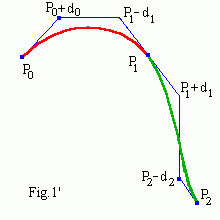# Interpolating cubic B-spline

## Bezier control points

B-spline does not interpolate its deBoor control points. As since Bezier curve goes through its terminal points therefore we will use Bezier control points for cubic uniform B-spline (really we use here only cubic Bezier splines joined C 2 smoothly).To determine the first cubic Bezier segment we could set its two terminal control points and tangents. Then the second segment is determined by the first point, two derivatives (continuous at the point) and the second control point. All the rest segments are determined in a similar way. It is more convinient to set terminal tangents of the whole spline curve. Fig.1' shows, that all Bezier control points of the curve are evaluated from two vectors (P0 , P1 , ... , Pn ) and (d0 , d1 , ... , dn ). Points (P0 , P1 , ... , Pn ), d0 , dn are set by user and d1 , d2 , ... , dn-1 are calculated.
 move add delete
Interactive interpolating cubic B-spline   Drag/Add/Delete control points by finger or mouse.

## Solving banded equations

It is evident that by definition (see Fig.1) the curve is C 1 continuous. From C 2 continuity it follows e.g.
P1 - 2(P1 - d1 ) + (P0 + d0 ) = (P2 - d2 ) - 2(P1 + d1 ) + P1 ,
d0 + 4d1 + d2 = P2 - P0 .

In general case we get banded 3-diagonals linear equations
4d1 + d2 = P2 - P0 - d0
d1 + 4d2 + d3 = P3 - P1
... ...
di + 4di+1 + di+2 = Pi+2 - Pi
... ...
dn-2 + 4dn-1 = Pn - Pn-2 - dn

We can successively express
di = Ai + Bi di+1 ,     i = 1 , 2 , ... , n-1
where
A1 = (P2 - P0 - d0 )/4 ,     B2 = -1/4,
Ai = (Pi+1 - Pi-1 - Ai-1 )/(4 + Bi-1 ),     Bi = -1/(4 + Bi-1 ).

Then we will find dn-1 and di successively by iterations in reverse order
dn-1 = An-1 + Bn-1 dn ,     di = ... .

It is programmed as (b-interpolate.js)

```function findCPoints(){
Bi = -.25;
Ax = (Px - Px - dx)/4;   Ay = (Py - Py - dy)/4;
for (var i = 2; i < N-1; i++){
Bi[i] = -1/(4 + Bi[i-1]);
Ax[i] = -(Px[i+1] - Px[i-1] - Ax[i-1])*Bi[i];
Ay[i] = -(Py[i+1] - Py[i-1] - Ay[i-1])*Bi[i]; }
for (var i = N-2; i > 0; i--){
dx[i] = Ax[i] + dx[i+1]*Bi[i];  dy[i] = Ay[i] + dy[i+1]*Bi[i]; }
}
```

## Handling the terminal tangents

If you do not like to worry about the terminal tangents of interpolating curve, you can direct them to the nearest point as for Cardinal curve, i.e. ("1/3 rule" is used)
d0 = (P1 - P0 )/3,     dn = (Pn - Pn-1 )/3.
 move add delete

Contents   Previous: Nonuniform B-splines   Next: NURBS
updated 21 August 2001